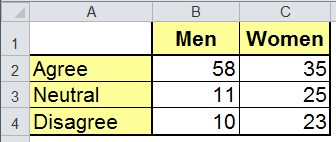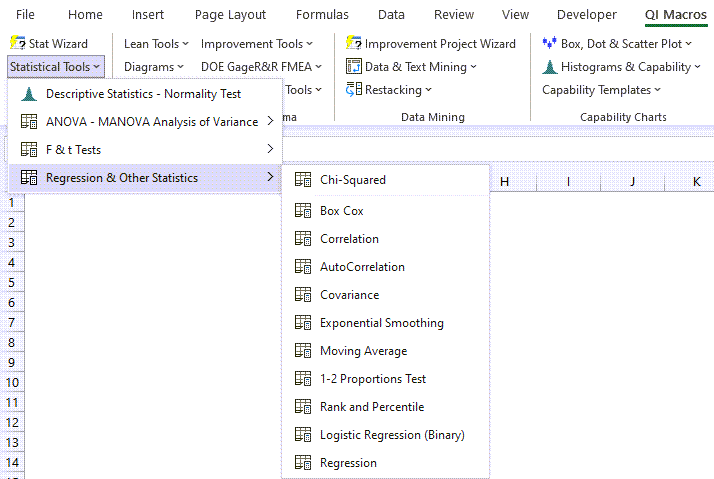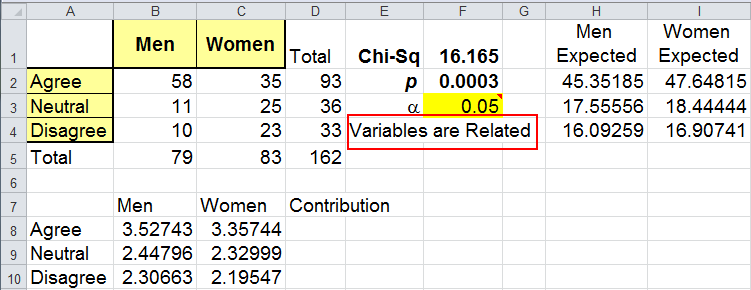# Want to Do a Chi-Square Test in Excel?

### There are different types of Chi Square tests:

Note: Excel does not do table tests; QI Macros adds this functionality.

## QI Macros can do Chi-Square Tables for you!

### Create Chi-Square Table using QI Macros:

2. Click on QI Macros menu > Statistical Tools > Regression > Chi-Square.
3. QI Macros will do the math and analysis for you.

### Go Deeper: Chi-Square test of a Contingency Table

A Chi Square test evaluates if two variables are independent of each other. We've all taken surveys and probably wondered what happened. A Chi Square test of a contingency table helps identify if there are differences between two or more demographics. Consider the following example.

### Men vs. Women - Chi Square test Example

We can develop a null hypothesis (H0) that point of view and gender are independent and an alternate hypothesis (Ha) that gender and point of view are related:

• H0 - two variables (gender and views) are independent
• Ha - two variables are dependent (views depend on gender)

Now, conduct the survey and enter the number of responses into Excel. As you can see, men seem to agree more than the women do, but is it statistically different?Select the data with the mouse and click on QI Macros Menu, Statistical Tools to select the one sample Chi Square test:The Chi Square test macro will calculate the values and interpret the results for you:Note that we don't need the same number of responses from each group to get a result.
Individual Chi-Square values are listed below the table. They add up to the total Chi-Square value in F1.

### Interpreting the Chi Square results

 If Then p value < a Reject the null hypothesis Variables are Related p value > a Cannot Reject the null hypothesis (Accept the null hypothesis) Variables are Independent

In the above results the p value is 0.00031.

If the p value is less than the significance (e.g., a=0.05), we can reject the null hypothesis that views are independent of gender. Gender and point of view are related; men and women have different points of view.

### Why Choose QI Macros Statistical Software for Excel?### Easy to Use

• Works Right in Excel
• Interprets p-values for You
• Accurate No-Worry Results
• Free Training Anytime### Proven and Trusted

• 100,000 Users in 80 Countries
• Celebrating 20th Anniversary
• Five Star CNET Rating - Virus Free### Affordable

• Only \$349 USD
Quantity Discounts Available
• No annual fees
• Free Technical Support